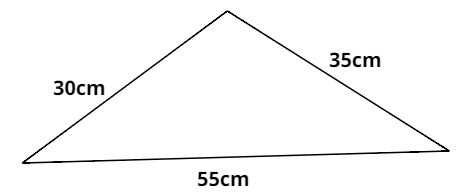QuestionAnswers

# Find the perimeter of the following triangle.Verified
$\therefore$Perimeter of the given triangle = 30 + 35 + 55 = 120 cm